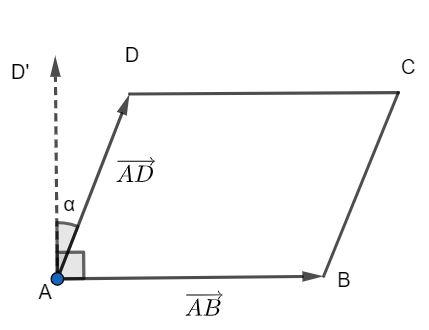QuestionAnswers

# Two adjacent sides of a parallelogram ABCD is given by $\overrightarrow {AB} = 2\hat i + 10\hat j + 11\hat k$ and $\overrightarrow {AD} = - \hat i + 2\hat j + 2\hat k$. The side AD is rotated by an acute angle $\alpha$ in the plane of the parallelogram so that AD becomes AD’. If AD’ makes a right angle with the side AB, then what is the cosine of the angle $\alpha$ ?(a). $\dfrac{8}{9}$(b). $\dfrac{{\sqrt {17} }}{9}$(c). $\dfrac{1}{9}$(d). $\dfrac{{4\sqrt 5 }}{9}$Verified
112.5K+ Views
Hint: Find the cosine of the angle between the vectors AB and AD using the formula $\cos \theta = \dfrac{{\overrightarrow {AB} .\overrightarrow {AD} }}{{|\overrightarrow {AB} ||\overrightarrow {AD} |}}$. This angle can be written as 90° – $\alpha$. Then find the value of $\sin \alpha$ and then $\cos \alpha$.The angle between two vectors a and b is given by the formula as follows:

$\cos \theta = \dfrac{{\overrightarrow a .\overrightarrow b }}{{|\overrightarrow a ||\overrightarrow b |}}$

The angle between the vectors AB and AD is given as follows:

$\cos \theta = \dfrac{{\overrightarrow {AB} .\overrightarrow {AD} }}{{|\overrightarrow {AB} ||\overrightarrow {AD} |}}$

The vectors are given by $\overrightarrow {AB} = 2\hat i + 10\hat j + 11\hat k$ and
$\overrightarrow {AD} = - \hat i + 2\hat j + 2\hat k$, hence, we have:

$\cos \theta = \dfrac{{(2\hat i + 10\hat j + 11\hat k).( - \hat i + 2\hat j + 2\hat k)}}{{\sqrt {{2^2} + {{10}^2} + {{11}^2}} \sqrt {{{( - 1)}^2} + {2^2} + {2^2}} }}$

Simplifying, we have:

$\cos \theta = \dfrac{{2( - 1) + 10.2 + 11.2}}{{\sqrt {4 + 100 + 121} \sqrt {1 + 4 + 4} }}$

$\cos \theta = \dfrac{{ - 2 + 20 + 22}}{{\sqrt {225} \sqrt 9 }}$

We know that the square root of 225 is 15 and the square root of 9 is 3.

$\cos \theta = \dfrac{{40}}{{15.3}}$

Simplifying, we have:

$\cos \theta = \dfrac{8}{9}............(1)$

From the figure, we know that $\theta$ is $90^\circ - \alpha$.

Then, in equation (1), we have:

$\cos (90^\circ - \alpha ) = \dfrac{8}{9}$

We know that the value of $\cos (90^\circ - x)$ is $\sin x$, then, we have:

$\sin \alpha = \dfrac{8}{9}............(2)$

We know that ${\cos ^2}x = 1 - {\sin ^2}x$, then using equation (2), we can find the value of $\cos \alpha$.

${\cos ^2}\alpha = 1 - {\sin ^2}\alpha$

${\cos ^2}\alpha = 1 - {\left( {\dfrac{8}{9}} \right)^2}$

Simplifying, we have:

${\cos ^2}\alpha = 1 - \dfrac{{64}}{{81}}$

Taking common denominator, we have:

${\cos ^2}\alpha = \dfrac{{81 - 64}}{{81}}$

${\cos ^2}\alpha = \dfrac{{17}}{{81}}$

Taking square root and retaining only the positive value, we have:

$\cos \alpha = \sqrt {\dfrac{{17}}{{81}}}$

Simplifying, we have:

$\cos \alpha = \dfrac{{\sqrt {17} }}{9}$

Hence, the correct answer is option (b).

Note: Always choose positive or negative root by the value of the trigonometric functions when the angle is in one of the four quadrants.